Chemistry Index

Lewis dot structures

 Lewis dot Structure The structure of carbon and its compound can be expressed using the Lewis-dot structure This system identifies how the atoms that compose a molecule of a specific compound are attached (bonded) to one another and (to some extent) oriented in space.  An atom is indicated by its symbol with a number of dots representing the number of valency electrons e.g Hydrogen would be H with a single dot, Carbon would be a C with four dots.   When two valency electrons are paired they are represented by two adjacent dots.   Paired valency electrons are not normally available for forming bonds with other atoms. Lewis diagrams are useful for visualizing both ionic and covalent bonds Outline rules for Lewis dot Structure 1. Determine whether the compound is covalent or ionic. Bonds   If covalent, treat the entire molecule.  If ionic, treat each ion separately.   Compounds of low electronegativity metals with high electronegativity (i.e With difference in electonegativity Values greater than 1,6 ) nonmetals are ionic as are compounds of metals with polyatomic anions.   For a monoatomic ion, the electronic configuration of the ion represents the correct Lewis structure.   For compounds containing complex ions, it is necessary to recognise the formulas of cations and anions. Ions and Cations 2. Determine the total number of valence electrons available to the molecule by examining the formula.   If there is a negative charge add one electron to the total for every negative charge.    If there is a positive charge indicated on the molecular formula subtract one electron for every positive charge indicated. Then divide the total number of available electrons by 2 to obtain the number of electron pairs (E.P.) available. 3. Organize the atoms so there is a central atom surrounded by (outer) atoms.The central atom usually has two or more valancy electrons.   Hydrogen is never the central atom as it only has one valency electron. 4. Arrange the central atoms and the other atoms distributed as symmetrical as possible around the central atoms and determine a provisional electron distribution by arranging the electron pairs (E.P.) in the following manner until all available pairs have been distributed: i) One pair between the central atom and outer atom. ii) Three additional pairs on each outer atom (except hydrogen, which has no additional pairs). This results in 4 E.P. (i.e., an octet) around each outer atom when the bonding pair is included in the count. iii) Remaining electron pairs (if any) on the central atom. 5) Using the valence electrons available from step 2, connect all the atoms together in one structure using bonding pairs symbolized by a dash for each bonding pair and double dots for each electron pair 6. Calculate the formal charge (F) on the central atom. a) Count the electrons shared as bonds. Total = b b) Count the electrons owned as lone pairs. Total = n c) F = V - (n + b/2), where V = number of valence electrons for the atom. 7. If the central atom formal charge is zero or is equal to the charge on the Formula, the provisional electron distribution from (4) is correct.   Calculate the formal charge of the outer atoms to complete the Lewis structure. 8. If the structure is not correct, calculate the formal charge on each of the outer atoms.   Then to obtain the correct structure, form a multiple bond by sharing an electron pair from the outer atom that has the most negative formal charge. a) For a central atom from the second (n = 2) row of the periodic table continue this process sequentially until the central atom has 4 E.P. (an octet). b) For all other elements, continue this process sequentially until the formal charge on the central atom is reduced to zero or two double bonds are formed. 9. Recalculate the formal charge of each atom to complete the Lewis structure. Typical Lewis dot Structures 1) Elements... The notes below relate to the arrangement of elements in the periodic table Periodic Table... All elements in Group 1 (alkali metals)represented by their elemental symbol with one dot. All elements in Group 2 called the alkali earth metals would have their electron dot structure represented by their respective elemental symbol with two dots. Group 13 Beginning with Boron would have three dots all separated, unpaired, and considered bonding electrons. Group 14 Topped by Carbon would have four dots representing four valence electrons all unpaired. Group 15 Starting with Nitrogen would have five dots two of which would be clustered together as a pair. Group 16 Beginning with Oxygen would have six valence electron consisting of two pairs of dots and two dots unpaired. Group 17 beginning with Fluorine would have seven valence electrons consisting of three pairs of dots and one single dot. Group 18 are the Inert Gas or better known as the Noble Gases is group 18. They would have eight electrons consisting of four pairs of dots.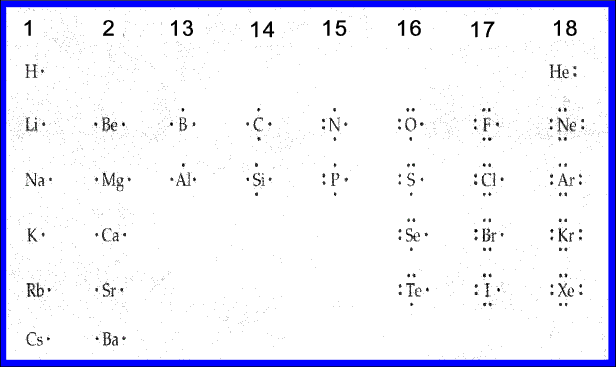2) Compounds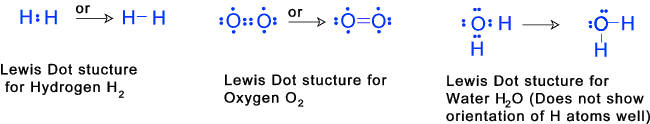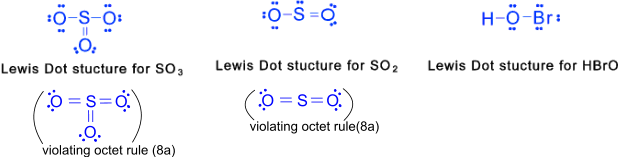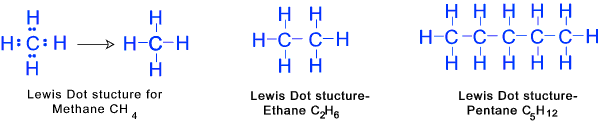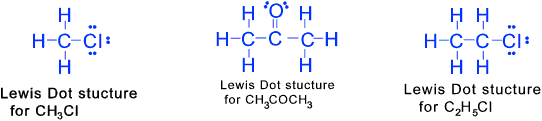Links relevant to organic chemistry.. Step by step procedures for constructing lewis dot structure.... Kent Chemistry Lewis Dot Structures ... Very clear diagrams for a variety of compounds. Hyperphysics Lewis dot structures of the elements... Very clear diagram of the structure of the elements Lewis Dot Structures... Set of rules and explanations... GENERAL CHEMISTRY CHAPTER FIVE...A set of notes which clearly explain the principles...
Chemistry Index